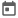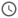# Verilog Syntax##### 10 Aug 2021##### 4 mins

Remembering syntax for any coding language is very vital for writing good codes. So let's look into the different Verilog syntax.

### Module

`module` keyword is used to declare a module which is the basic building block of verilog code. A module always have a associated `endmodule` keyword, which marks end of the module.

``````// A full adder module having A, B & Cin as the input
// and sum & carry as output
module fullAdder(input A, B, Cin, output sum, carry)
...
...
...
endmodule``````

Comments are important part for professional coding as it increases readability of the code. In Verilog, there are 2 types of comment.

1. Single-line comment — `//` is used to write single line comment.

2. Multi-line comment — This is used to write comments which extends to more than 1 line. Multi line comments start with `/*` and ends with `*/`. Everything between these 2 keywords is treated as comment.

### Operators

#### Operands

The variable upon which the operation is done is known as operand.

#### Different Operators

Operators are used to create expressions. Operators are basically of 3 types depending upon the number of operands it has.

1. Unary Operator — It has only one operand. Ex - `x = ~a`
2. Binary Operator — It has 2 operands. Ex - `x = a + b`
3. Ternary Operator — It has 3 operands. It acts as a replacement of if-else. Ex - `x = a ? b : c`. This means that if `a` is true, i.e., 1 then `x` will be assigned value of `b`, otherwise value of `c` will be assigned to `x`.

### Number Literals

As Verilog represents a hardware and in hardware different base numbers are used, thus Verilog supports different type of number systems base. Also, the way of writing a number literal is different in Verilog. Also, hardware can work even on single bit data or even on 32- or 64-bit data, thus for every number literal we need to provide the number of bits.

This is the syntax to write a number literal in Verilog.
`[no_of_bits]’[base][numerical_value]`
Where,
no_of_bits = size of the literal
base = base of the number, it can take these values `b` or `B` for binary, `o` or `O` for octal, `d` or `D` for decimal, and `h` or `H` for hexadecimal
numerical_value = value of the number in the specified base

#### Example

``````module xyz;
// ...
// all numerical value evaluates to 179 in decimal base
a = 8'b10110011;// 8-bit binary number
b = 8'o263;     // 8-bit octal number
c = 8'd179;     // 8-bit decimal number
d = 8'hb3;      // 8-bit hexadecimal number
endmodule``````

### String Literals

Any literal between `" "` is treated as string by Verilog. Strings can be thought of as array of characters. Verilog uses ASCII to store the characters and thus each character requires 8-bit or 1-byte.

String literals cannot be written in multiple lines.

#### Example

``````module xyz;
// ...
a = " erdd " // String of 6 characters
b = "this is // Invalid string literal
awsomwe"
endmodule``````

### Identifiers

Identifiers are any text which is used as a variable name. These text or strings are used to identify a particular variable, module, etc.

In Verilog there are some rules which needs to be followed for identifiers:

1. Identifiers can only contain `[0-9][A-Z][a-z]` and `_` or `\$`.
2. Identifier should not start with `\$` or `[0-9]`
3. Identifier should not contain only numbers.
4. Identifier should not contain any white spaces.
5. Identifiers are case sensitive. That means `half_adder` and `Half_adder` are 2 different identifiers.
6. Keywords cannot be used as identifiers.

#### Example

``````module xyz;
// ...
reg ab\$23 = 0;  // Valid Identifer
reg ab_12 = 0;  // Valid Identifer
reg _a = 0;     // Valid Identifer
reg 23ec = 0;   // Invalid Identifer
reg 12 = 0;     // Invalid Identifier
reg as@12 = 0;  // Invalid Identifier
reg as 23 = 0;  // Invalid identifier
endmodule``````

### Keywords

Keywords are special literals which has certain meaning to Verilog language. These keywords should not be used as identifiers, as Verilog will interpret it as keywords.

Some of the keywords are: